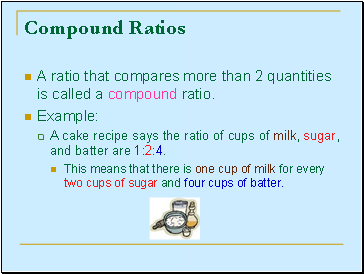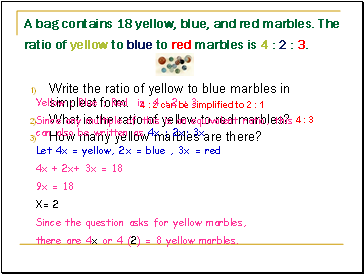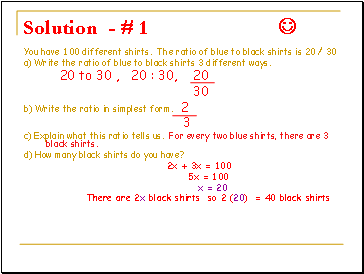# RatioPage 2

#### WATCH ALL SLIDES

4 : 6 (multiply original ratio by by 2)

6 : 9 (multiply original ratio by by 3)

8 : 12 (multiply original ratio by by 4)

The ratio 2 : 3 can be expressed as

2x to 3x (multiply the original ratio by any number x)

Slide 11## Compound Ratios

A ratio that compares more than 2 quantities is called a compound ratio.

Example:

A cake recipe says the ratio of cups of milk, sugar, and batter are 1:2:4.

This means that there is one cup of milk for every two cups of sugar and four cups of batter.

Slide 12A bag contains 18 yellow, blue, and red marbles. The ratio of yellow to blue to red marbles is 4 : 2 : 3.

Write the ratio of yellow to blue marbles in simplest form.

What is the ratio of yellow to red marbles?

How many yellow marbles are there?

4 : 2 can be simplified to 2 : 1

4 : 3

Yellow : Blue : Red is 4 : 2 : 3

Since any multiple of this is an equivalent ratio, this can also be written as 4x : 2x: 3x

Let 4x = yellow, 2x = blue , 3x = red

4x + 2x+ 3x = 18

9x = 18

X= 2

Since the question asks for yellow marbles,

there are 4x or 4 (2) = 8 yellow marbles.

Slide 13## Practice problem

# 1

(1) You have 100 different shirts. The ratio of blue to black shirts is 20 .

30

a) Write the ratio of blue to black shirts 3 different ways.

b) Write the ratio in simplest form.

c) Explain what this ratio tells us.

d) How many black shirts do you have?

Slide 14Solution - # 1 

You have 100 different shirts. The ratio of blue to black shirts is 20 / 30

a) Write the ratio of blue to black shirts 3 different ways.

20 to 30 , 20 : 30, 20

30

b) Write the ratio in simplest form. 2

3

c) Explain what this ratio tells us. For every two blue shirts, there are 3 black shirts.

d) How many black shirts do you have?

2x + 3x = 100

5x = 100

x = 20

There are 2x black shirts so 2 (20) = 40 black shirts

Slide 15Practice Word Problems

You go to a party where the ratio of boys to girls is 28 to 56. Express the ratio of boys to girls in simplest form.

Explain what this ratio tells us.

28 / 56 = 1 / 2

The ratio of boys to girls is 1 to 2

(2) For every 1 boy there are 2 girls at the party.

Slide 16## Practice Word Problems

Mindy has 72 candy bars. If the ratio of Mars to Snickers is 8:4, Find the number of each type of candy.

Go to page:
1  2  3